Squares, roots and powers

This free course is available to start right now. Review the full course description and key learning outcomes and create an account and enrol if you want a free statement of participation.

Free course

3.2.1 Try some yourself

Activity 37

Use the method outlined in Example 9 to estimate each of the following, and then use your calculator to evaluate each, giving your answers to six significant figures.

• (a) 2521 ÷ 38

• (b) 17.85 × 286.3

• (c) 1452 ÷ 0.0072

• (d) 0.0053 × 0.0078 ÷ 0.6821

• (e) 0.000 923 × 0.007 67.

• (a) Estimate: 2521 = 2.521 × 1033 × 103

•    38 = 3.8 × 1014 × 101.

• So 2521 ÷ 38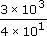=× 103−1

• = 0.75 × 102 = 75.

• Calculate: 2521 ÷ 38 = 66.3421 rounded to six significant figures.

• (b) Estimate: 17.85 = 1.785 × 1012 × 101

•   286.3 = 2.863 × 1023 × 102.

• So 17.85 × 286.32 × 101 × 3 × 102

• = 2 × 3 × 101 × 102 = 6 × 103 = 6000.

• Calculate: 17.85 × 286.3 = 5110.46 rounded to six significant figures.

• (c) Estimate: 1452 = 1.452 × 1031.4 × 103

•    0.0072 = 7.2 × 10-37 × 10−3.

• (Notice that in this case it is easier to round to 1.4 than to 1 or 1.5, anticipating the next step, since it is easier to find 1.4 ÷ 7 than 1 ÷ 7 or 1.5 ÷ 7.)

• So 1452 ÷ 0.0072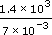=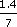× 103–3

• = 0.2 × 103+3 = 0.2 × 106 = 2 × 10−1 × 106

• = 2 × 10−1+6 = 2 × 105 = 200 000.

• Calculate: 1452 ÷ 0.0072 = 201 667 rounded to six significant figures.

• (d) Estimate: 0.0053 = 5.3 × 10−35 × 10−3

• 0.0078 = 7.8 × 10−38 × 10−3

• 0.6821 = 6.821 × 10−17 × 10−1.

• So

•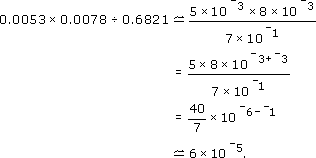• Calculate: 0.0053 × 0.0078 ÷ 0.6821

• = 6.06069 × 10−5 = 0.000 060 6069 rounded to six significant figures.

• (Notice that the calculator gives the answer in scientific notation.)

• (e)

0.000 923 × 0.007 67 = 9.23 × 10−4 × 7.67 × 10−3

• Estimate:

•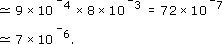• Calculate:

•   0.000 923 × 0.007 67 = 7.079 41 × 10−6.

Activity 38

A light year is the distance light travels through space in one year. The centre of the Milky Way galaxy is 2.6 × 104 light years away from Earth. One light year is 9.46 × 1012 km. How far away is the centre of the Milky Way in kilometers? Estimate your answer first and then use your calculator to do the calculation, giving your answer in scientific notation.

Estimate: The centre is roughly 3 × 104 light years away, and each light year is roughly 9 × 1012 km, so the distance to the centre is approximately 3 × 104 × 9 × 1012 = 27 × 1016 = 2.7 × 1017.

Calculate:

2.6 × 104 × 9.46 × 1012 = 2.4596 × 1017.

MU120_4M4